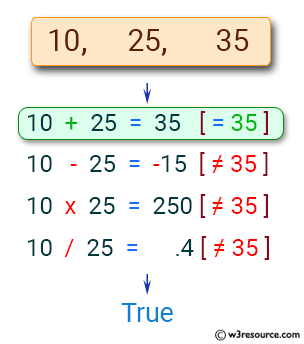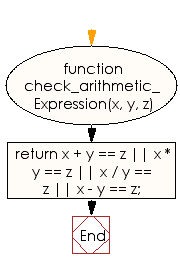# JavaScript: Check whether it is possible to replace \$ in a specified expression

## JavaScript Basic: Exercise-89 with Solution

Write a JavaScript program to check whether it is possible to replace \$ in a given expression x \$ y = z with one of the four signs +, -, * or / to obtain a correct expression.

For example x = 10, y = 30 and z = 300, we can replace \$ with a multiple operator (*) to obtain x * y = z

Pictorial Presentation:Sample Solution:

HTML Code:

``````<!DOCTYPE html>
<html>
<meta charset="utf-8">
<meta name="viewport" content="width=device-width">
<title>Check whether it is possible to replace \$ in a specified expression</title>
<body>

</body>
</html>
```
```

JavaScript Code:

``````function check_arithmetic_Expression(x, y, z) {
return x + y == z || x * y == z || x / y == z || x - y == z;
}

console.log(check_arithmetic_Expression(10, 25, 35))
console.log(check_arithmetic_Expression(10, 25, 250))
console.log(check_arithmetic_Expression(30, 25, 5))
console.log(check_arithmetic_Expression(100, 25, 4.0))
console.log(check_arithmetic_Expression(100, 25, 25))
```
```

Sample Output:

```true
true
true
true
false
```

Flowchart:ES6 Version:

``````function check_arithmetic_Expression(x, y, z) {
return x + y == z || x * y == z || x / y == z || x - y == z;
}

console.log(check_arithmetic_Expression(10, 25, 35))
console.log(check_arithmetic_Expression(10, 25, 250))
console.log(check_arithmetic_Expression(30, 25, 5))
console.log(check_arithmetic_Expression(100, 25, 4.0))
console.log(check_arithmetic_Expression(100, 25, 25))
``````

Live Demo:

See the Pen javascript-basic-exercise-89 by w3resource (@w3resource) on CodePen.

What is the difficulty level of this exercise?

Test your Programming skills with w3resource's quiz.

﻿

## JavaScript: Tips of the Day

Converts a string to title case

Example:

```const toTitleCase = str =>
str
.match(/[A-Z]{2,}(?=[A-Z][a-z]+[0-9]*|\b)|[A-Z]?[a-z]+[0-9]*|[A-Z]|[0-9]+/g)
.map(x => x.charAt(0).toUpperCase() + x.slice(1))
.join(' ');
console.log(toTitleCase('some_database_field_name')); // 'Some Database Field Name'
console.log(toTitleCase('Some label that needs to be title-cased')); // 'Some Label That Needs To Be Title Cased'
console.log(toTitleCase('some-package-name')); // 'Some Package Name'
console.log(toTitleCase('some-mixed_string with spaces_underscores-and-hyphens')); // 'Some Mixed String With Spaces Underscores And Hyphens'
```

Output:

```"Some Database Field Name"
"Some Label That Needs To Be Title Cased"
"Some Package Name"
"Some Mixed String With Spaces Underscores And Hyphens"
```